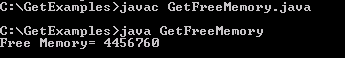Tutorials

# Java get Free Memory

In this section, we are going to obtain the free memory. In order to get the memory left of being allocated, the method getruntime() returns the runtime object associated with the Java application.

In this section, we are going to obtain the free memory. In order to get the memory left of being allocated, the method getruntime() returns the runtime object associated with the Java application.

# Java get Free Memory

In this section, we are going to obtain the free memory. In order to get the memory left of being allocated, the method getruntime() returns the runtime object associated with the Java application.

freeMemory- This method returns the amount of free memory.

Here is the code of GetFreeMemory.java

 ``` public class GetFreeMemory {   public static void main(String[]args){   long free = Runtime.getRuntime().freeMemory();   System.out.println("Free Memory= "+free);   } }```

Output will be displayed as: